# Logical Reasoning for Placement Test - Set 2

1)   In the following question, choose the correct code form.

If, in a language, ‘one’ is called ‘two’, ‘two’ is called ‘three’, ‘three’ is called ‘four’, ‘four’ is called ‘five’ and ‘five’ is called ‘six’.
Then what is the square of number 2?

a. Three
b. Four
c. Five
d. Six
e. None of these
 Answer  Explanation ANSWER: Five Explanation: The square of 2 is 4.But four is called five in this language. So, the correct answer is C.

2)   If ‘dog’ is called ‘lion’, ‘lion’ is called ‘bison’, ‘bison’ is called ‘snake’, ‘snake’ is called ‘mongoose’, ‘mongoose’ is called ‘crocodile’, then which one is reared as pet?

a. Lion
b. Bison
c. Snake
d. Mongoose
e. None of these
 Answer  Explanation ANSWER: Lion Explanation: Generally, dog is reared as pet.But dog is called lion, so ‘lion’ is reared as pet.

3)   Directions: A team of 5 players Ashwin, Rahul, Sachin, Virat and Rohit participated in a tournament and played four matches.

The following table gives partial information about their individual scores and the total runs scored by the team in each match.

Each column has two values missing. These are the runs scored by the two lowest scorers in that match.

None of the two missing values is more than 10% of the total runs scored in that match.

If the absolute difference between the total runs scored by Ashwin and Sachin in the four matches is minimum possible.

What is the absolute difference between total runs scored by Rahul and Rohit in the four matches?
Runs scoredMatch 1Match 2Match 3Match 4
Ashwin10053
Rahul886552
Sachin110
Virat72752056
Rohit6078
Total270300240200

a. 27
b. 32
c. 37
d. 42

Explanation:
Maximum possible total runs scored by Sachin in the four matches = 27 + 30 + 110 + 20 = 187.

You can see that we have again taken 10% values in each match.

Completing the table:

Runs scoredMatch 1Match 2Match 3 Match 4
Ashwin231001353
Rahul88651952
Sachin273011020
Virat72752056
Rohit60307819
Total270300240200
In such a case, minimum possible total runs scored by Ashwin in the four matches = 23 + 100 + 13 + 53 = 189.

Difference = 189 – 187 = 2 (minimum possible)

To minimize the difference, we have taken minimum possible score of Ashwin. Hence, his score in Match-3 is taken as 13.

Subsequently, total runs scored by Rahul in the four matches = 88 + 65 + 19 + 52 = 224.
Also, total runs scored by Rohit in the four matches = 60 + 30 + 78 + 19 = 187

Absolute difference = 224 – 187 = 37

4)   Choose the word which is least like the other words in the group.

a. Timor
b. India
c. Rhodes
d. Borneo
 Answer  Explanation ANSWER: India Explanation: All others are islands. India is a peninsula.

5)   Choose the word which is not similar to the other words in the group.

a. Bonnet
b. Fender
c. Dashboard
d. Hubcap
e. Quay
 Answer  Explanation ANSWER: Quay Explanation: All others are parts of a car. Quay is the odd one out.

• Five brothers are standing in a row facing North.
• Tony is not adjacent to Bony or Mony. Sony is not adjacent to Bony. Tony is adjacent to Dony. Dony is at the middle in the row.
Then, which pair is at the extreme ends?

a. Tony, Dony
b. Dony, Bony
c. Sony, Mony
d. Mony, Tony

Explanation:
If a person is facing North, then “Your left and right” will be the same as the “Person facing North”

Dony is the middle i.e. _____, _____, Dony, _____, _____

Tony is adjacent to Dony i.e. _____, Tony, Dony ____, ______

Tony is not adjacent to Bony or Mony i.e.

_____, Tony, Dony, Bony, Mony

So, the sequence becomes - Sony, Tony, Dony, Bony, Mony

## Sony Tony Dony Bony Mony

• There are seven monkeys sitting in a row -  Annie, Bonnie, Connie, Donnie, Earnie, Fernie and Genie.
• Genie is to the right of Donnie and to the left of Bonnie.
• Annie is on the right of Connie.
• Annie and Donnie have one monkey between them.
• Earnie and Bonnie have two monkeys between them.
• Donnie and Fernie have two monkeys between them.
Who is on the immediate right of Bonnie?

a. Donnie
b. Earnie
c. Genie
d. Fernie
 Answer  Explanation ANSWER: Fernie Explanation: Genie is to the right of Donnie and to the left of Bonnie i.e. Donnie, Genie, Bonnie.Annie is on the right of Connie i.e. Connie, AnnieEarnie and Bonnie have two monkeys between them i.e. Connie, Annie, Earnie, Donnie, Genie, Bonnie.Since Donnie and Fernie have two monkeys between them, the sequence becomes – Connie, Annie, Earnie, Donnie, Genie, Bonnie, Fernie.Connie Annie Earnie Donnie Genie Bonnie Fernie

8)   325, 259, 204, 160, 127, ?, 94

a. 94
b. 105
c. 110
d. 123
 Answer  Explanation ANSWER: 105 Explanation: The pattern in the given series - -66, -55, -44, -33, -22, ……The missing number should be: 127 – 22 = 105

9)   1, 4, 8, 13, ?, 26

a. 15
b. 19
c. 20
d. 21
 Answer  Explanation ANSWER: 19 Explanation: The pattern in the given series is - +3 +4 +5 +6 +7 ……The next term should be: 13 + 6 = 19

10)   In the question below is given a statement followed by two courses of action numbered I and II. You have to assume everything in the statement to be true and based on the information given in the statement, decide which of the suggested courses of action logically follow(s) for pursuing.

(A) If only I follows
(B) If only II follows
(C) If either I or II follows
(D) If neither I nor II follow
(E) If both I and II follow.

Statement - Tribunals take too long in deciding disputes of various departments.

Courses of Action - I.  Tribunals should be ordered to speed up matters.

II. Special powers should be granted to Head of Departments to settle disputes concerning their departments.

a. Only I follows
b. Only II follows
c. Either I or II follows
d. Neither I nor II follow
e. Both I and II follow
 Answer  Explanation ANSWER: Both I and II follow Explanation: 1) The work in the tribunals needs to be speeded up.2) The system needs to be reorganised so that more number of problems can be resolved at the departmental level itself, to provide justice to the people. So, both courses follow.

11)   In the question below is given a statement followed by two courses of action numbered I and II. You have to assume everything in the statement to be true and based on the information given in the statement, decide which of the suggested courses of action logically follow(s) for pursuing.

(A) If only I follows
(B) If only II follows
(C) If either I or II follows
(D) If neither I nor II follow
(E) If both I and II follow.

Statement - Every year, we have cases of dengue at the end of rainy season, but it turned out to be an epidemic this year.

Courses of Action - I. Every five years, vaccination drive should be organised.

II. Awareness should be spread among people get rid of the rubbish in their surroundings.

a. Only I follows
b. Only II follows
c. Either I or II follows
d. Neither I nor II follow
e. Both I and II follow
 Answer  Explanation ANSWER: Only II follows Explanation: The disease occurs at the end of monsoons every year. So, precautionary measures every five years shall not help. The second method is a preventive measure and should be followed by all the people. So, only II follows.

12)   In the question below is given a statement followed by two conclusions numbered I and II.

(A) If only I follows
(B) If only II follows
(C) If either I or II follows
(D) If neither I nor II follows
(E) If both I and II follow.

Statement - Cactus plants have fleshy stem and require little water.

Conclusions - I. All fleshy plants require less amount of water.

II. Cactus plants may be grown in places where water is not in abundance.

a. Only I follows
b. Only II follows
c. Either I or II follows
d. Neither I nor II follows
e. Both I and II follow
 Answer  Explanation ANSWER: Only II follows Explanation: In this case, statement is made only about Cactus plants, not all plants. Hence, I does not follow.Since, Cactus plants require less amount of water, they can be grown in places where water is not in abundance. So, II follows.

13)   In the question below is given a statement followed by two conclusions numbered I and II.

(A) If only I follows
(B) If only II follows
(C) If either I or II follows
(D) If neither I nor II follows
(E) If both I and II follow.

Statement - Adversity makes a man wise.

Conclusions - I. The poor are wisest in the world.

II. One must learn from their experience.

a. Only I follows
b. Only II follows
c. Either I or II follows
d. Neither I nor II follows
e. Both I and II follow
 Answer  Explanation ANSWER: Only II follows Explanation: The statement given here does not speak of money or riches.It only speaks of ‘adversity’ in general. So, I does not follow.Course II correctly relates to the given statement, so, II follows.

• Sam walks 20 m in front and 20 m to the right.
• Then every time turning to his left, he walks 10, 30, 30 m respectively.
How far is he now from his starting point?

a. 10 m
b. 20 m
c. 30 m
d. 40 m
 Answer  Explanation ANSWER: 10 m Explanation: The movement of Sam is as shown in the figure(O to P, P to Q, Q to R, R to S and S to T)Since, TS = OP + QRSo, T lies in line with OOT = RS – PQ = 30 – 20 = 10 m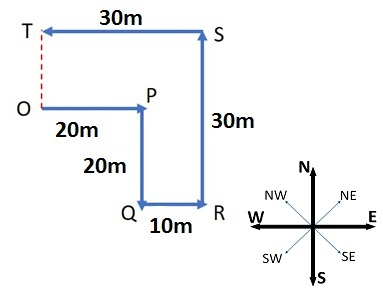15)   Niranjan moved a distance of 150 m towards north. He then turned to the left and walked for about 50 m, turned left again and walked another 160 m. Finally, he turned to the right at an angle of 45°?

In which direction was he moving finally?

a. South-west
b. South-east
c. North-west
d. North-east
 Answer  Explanation ANSWER: South-west Explanation: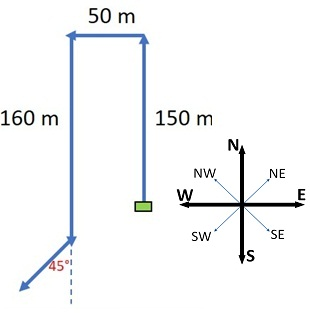16)   Pointing to a man, Rohan said, “His only brother is the father of my daughter’s father.” How is the Rohan related to the man?

a. Father
b. Grandson
c. Uncle
d. Nephew
 Answer  Explanation ANSWER: Nephew Explanation: Father of Rohan’s daughter’s father - Rohan’s father.So, the man’s brother is Rohan’s father or the man is the brother of Rohan’s father i.e. Rohan’s uncle.Rohan is the nephew of the man.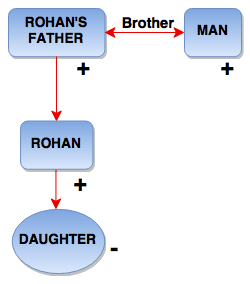17)   Pointing towards a girl, Amit said, “She is the daughter of only son of my grandfather”. How is the Amit related to the girl?

a. Brother
b. Uncle
c. Cousin
d. Father
 Answer  Explanation ANSWER: Brother Explanation: Grandfather’s only son – Amit’s fatherAmit’s father’s daughter – Amit’s sister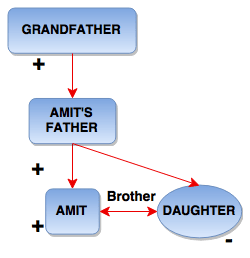18)   A correct clock shows 9 o'clock in the morning. Through how many degrees will the hour hand rotate when the clock shows 3 o'clock in the afternoon?

a. 144°
b. 150°
c. 160°
d. 180°
 Answer  Explanation ANSWER: 180° Explanation: Hour hand traverses 30° per hour.For 6 hours, 6 x 30 = 180°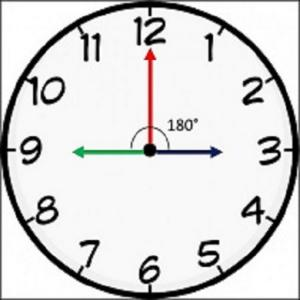19)   At what angle are the hands of a clock inclined at 15 minutes past 4?

a. 32°
b. 37°
c. 32.5°
d. 37.5°
 Answer  Explanation ANSWER: 37.5° Explanation: At 4 o’ clock, the hour hand is at 120° from the vertical. In 15 minutes,Hour hand = 120 + 15 x (0.5) = 120 + 7.5 = 127.5° (The hour hand moves at 0.5 dpm)Minute hand = 15 x (6) = 90° (The minute hand moves at 6 dpm)Difference or Angle between the hands = 127.5 – 90 = 37.5°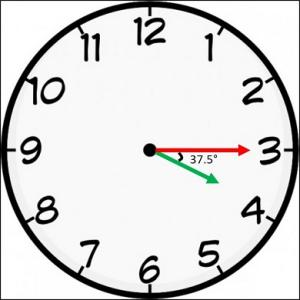20)   Rohit has a watch that gains 5 seconds in 3 minutes. It was set right at 9 a.m. in the morning.

In the afternoon of the same day, when the watch indicated 4 o'clock, how much time will the watch have gained?

a. No change
b. 10 min
c. (11)2/3 min
d. 12 min
 Answer  Explanation ANSWER: (11)2/3 min Explanation: Time from 9 a.m. to 4 p.m. = 7 hrsThe clock gains 5 secs in 3 minIn 60 min, it will gain, (60 x 5) / 3 = 100 seci.e. the clock gains 100 seconds in one hourIn 7 hours, it will gain 700 sec.700/60 mins = (11)2/3 mins